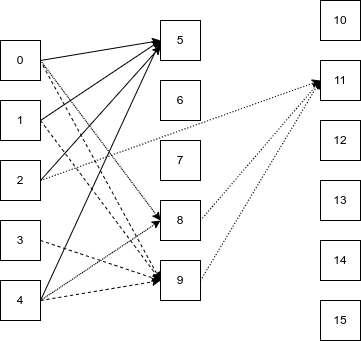# Algorithm for sorting graph nodes to increase cache locality

I have a directed acyclic graph with millions of nodes. The graph is split up in dependency layers, so that the nodes of layer n is only linked to by nodes from layers 1 to n-1.

The value of each node is calculated from incoming nodes. The value stored in a node is small, say maximum 8 bits.When the values at layer 0 of the graph chance, the whole graph is recalculated in stages, from left to right.

For each layer, the nodes are recalculated in index order: first node 5, then node 6 etc.

As you can see in the graph above, node 5 is dependent on nodes 0, 1, 2, and 4. Similarly, node 9 depends on values 0, 1, 3, and 4.

For cache locality, it would be much better to change the index of node 9 to 6, so that the values stored in 0, 1, and 4, used by node 5, are still warm in the cache when node 6 is calculated.

Of course, swapping the positions of nodes 9 and 6 may result in a less optimal pattern for what was originally node 6.

The work that is done in the shader is minimal, so the whole graph update operation is heavily memory bound.

What I’m looking for is for suggestions on algorithms to sort my graph for improved cache locality.